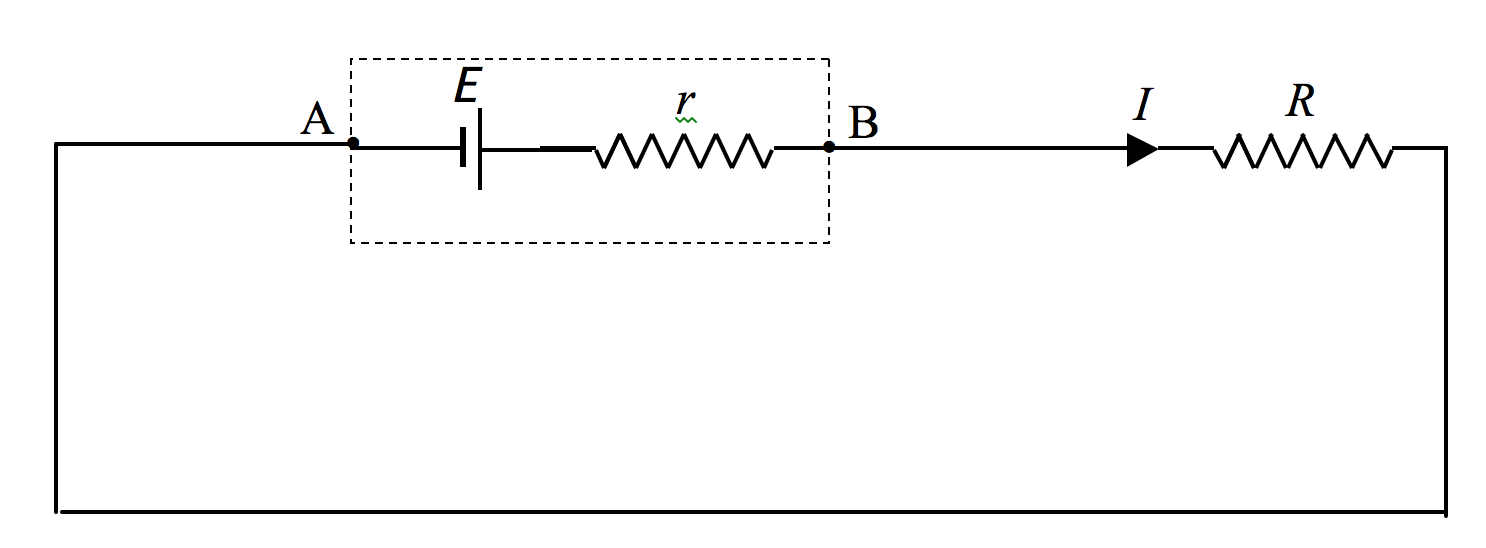$$\require{cancel}$$

4.7: Electromotive Force and Internal Resistance

•• Contributed by Jeremy Tatum
• Emeritus Professor (Physics & Astronomy) at University of Victoria

The reader is reminded of the following definition from section 4.1:

Definition. The potential difference across the poles of a cell when no current is being taken from it is called the electromotive force (EMF) of the cell.

I shall use the symbol E for EMF.

Question. A 4 $$\Omega$$ resistance is connected across a cell of EMF 2 V. What current flows?

The immediate answer is 0.5 A – but this is likely to be wrong. The reason is that a cell has a resistance of its own – its internal resistance. The internal resistance of a lead-acid cell is typically quite small, but most dry cells have an appreciable internal resistance. If the external resistance is $$R$$ and the internal resistance is $$r$$, the total resistance of the circuit is $$R + r$$, so that the current that flows is E$$/(R + r)$$.

Whenever a current is taken from a cell (or battery) the potential difference across its poles drops to a value less than its EMF. We can think of a cell as an EMF in series with an internal resistance:$$\text{FIGURE IV.4}$$

If we take the point A as having zero potential, we see that the potential of the point B will be E - $$Ir$$, and this, then, is the potential difference across the poles of the cell when a current $$I$$ is being taken from it.

Exercise

Show that this can also be written as $$\frac{ER}{R+r}$$.